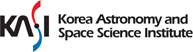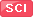Journal Paper

전체
The Pulsating Eclipsing Binary TIC 309658221 in a 7.59-day Orbit• Lee, Jae Woo; Martti H. Kristiansen; Kyeongsoo Hong;
• 2019-06-01
• ASTRONOMICAL JOURNAL 157 6 : 223-1~223-8
We present a new eclipsing binary (EB) showing multiperiodic oscillations using the first three sectors of Transiting Exoplanet Survey Satellite (TESS) photometry. The eclipse and pulsation light curves of TIC 309658221 were modeled using an iterative method to obtain a consistent photometric solution. The TESS target is a circular-orbit, detached binary system with a mass ratio of 0.349, an inclination angle of 80fdg42, and a temperature difference of 847 K between the components. The primary component of the system lies near the red edge of the δ Sct instability region on the main-sequence band in the Hertzsprung？Russell diagram. Multiple frequency analyses were applied to the eclipse-subtracted residuals after removing the binary effects in the observed data. These resulted in the detection of 26 frequencies, of which ${f}_{1}-{f}_{6}$ were independent pulsation frequencies. The 20 other frequencies could be mainly caused by orbital harmonics (f 8 and f 11) or combination frequencies. The period ratios and pulsation constants of the ${f}_{1}-{f}_{6}$ frequencies are in the ranges of ${P}_{\mathrm{pul}}/{P}_{\mathrm{orb}}=0.010\mbox{--}0.013$ and Q =0.027？0.036 days, respectively, which are typical of δ Sct type. The results reveal that TIC 309658221 is an eclipsing δ Sct star with an orbital period of 7.5952 days and pulsation frequencies of 9.94？13.01 day？1. This work demonstrates that the two-minute cadence observations of TESS are very useful for the study of pulsating EBs with multiple frequencies and low amplitudes.